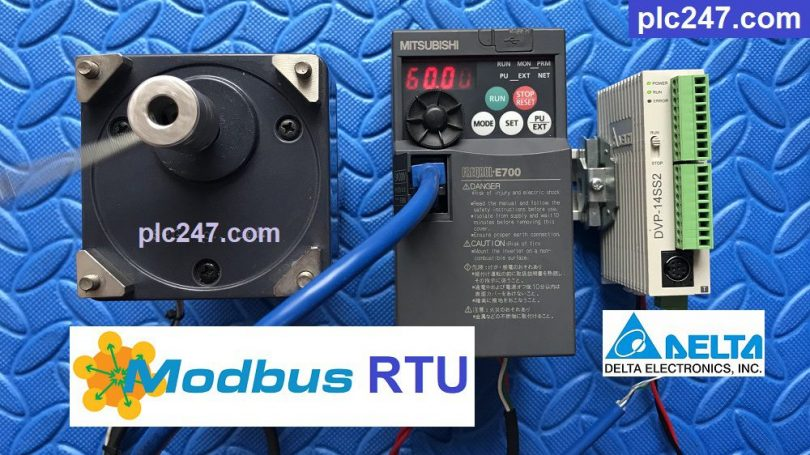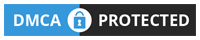# DELTA DVP 14SS2 “Modbus RTU” FR E720 Mitsubishi VFDWritten by

Hello everyone. PLC Delta SVP-Series is a type of PLC originating from Taiwan, it is very cheap and has high quality, programming like Mitsubishi or Siemens PLC optionally, so it is extremely popular in many applications. small machines.

Today plc247.com will start a series of articles to guide you to use PLC Delta DVP-14SS2 to control inverters and thermostats via Modbus-RTU. And today I use it to control Mitsubishi FR-E720 Inverter.

### Mitsubishi FR-E700 Configurator

In addition to the motor settings such as frequency, current, and voltage, we set the communication parameters as follows:

+ Pr117 (Slave Address) = 1

+ Pr118 (Baudrate) = 3 (9600bps)

+ Pr119 = 0 (8bit, stop bit =1)

+ Pr120 = 2 (Even)

+ Pr121 = 9999

+ Pr122 = 9999

+ Pr123 = 9999

+ Pr124 = 1

+ Pr340 = 1

+ Pr549 = 1

+ Pr79 = 2 (Last setting)

With inverter FR-E700 Series

>>> Control Address = 40009 – 40001 = 8 (dec) = 8 (hex)

• Set Word 8(hex) = 0000 0000 0000 0010 = 2 >>> Forward Run
• Set Word 8(hex) = 0000 0000 0000 0100 = 4 >>> Reverse Run
• Set Word 8(hex) = 0000 0000 0000 0000 = 0 >>> Motor Stop

>>> Set Frequency Address =40014 – 40001 = 13 (dec) = D (hex)

+ Output Frequency = 40201 – 40001 = 200 (dec) = C8 (hex)

+ Output Current = C9 (hex)

+ Output Voltage =CA (hex)

#### DELTA DVP 14SS2 Modbus RTU Configuration

You follow the image below to configure the communication:

### DVP 14SS2 PLC Programming

+ Modbus-RTU Configuration (Self-generated software via Modbus configuration)

+ Frequency Setting Command

+ Motol Control Command

+ Data Monitoring

### Detailed Instruction Video

======

Software & Documention Reference

+ Mitsubishi FR-E720 Manual PDF (Password Extract: plc247.com)